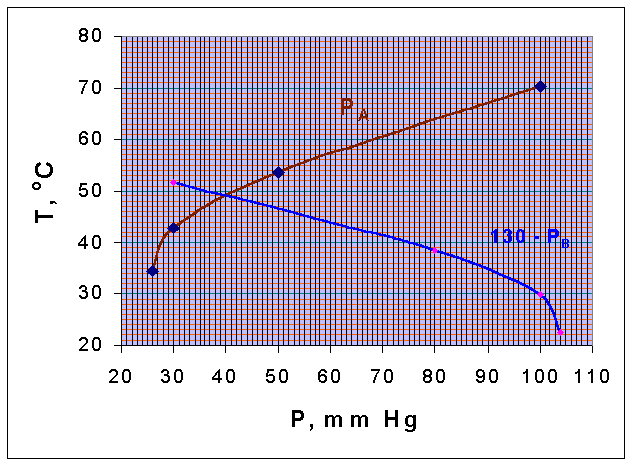### Steam Distillation

Vapor pressures of chlorobenzene and water are given below:

 Pressure, mm Hg 100 50 30 26 Temperature oC, Chlorobenzene 70.4 53.7 42.7 34.5 Temperature oC, Water 51.7 38.5 29.9 22.5

If steam is blown into the still containing a mixture of these two components and the total pressure is 130 mm Hg, estimate the temperature of boiling and the composition of the distillate. The two components are immiscible in the liquid

Calculations:

A mixture consisting of two immiscible components will boil at a temperature, at which the sum of their vapor pressure equal the total pressure.

i.e., at boiling point,

PA + PB = PT

Let chlorobenzene is taken as A, and water as B.

TA vs PA and TB vs (130 - PB) curves are drawn.

These two curves intersect when, PA = (130 - PB). (that is, when the total pressure is = 130 mm Hg)From the diagram it could be seen that the intersection point corresponds to a temperature of 49oC. This is the boiling point of the mixture. The vapor pressure of A and B at these temperature are obtained from the graph, as

PA = 40.25 mm Hg

PB = 130 - 40.25 = 89.75 mm Hg

Composition of distillate = 100 x (PA/PT)= 100 x (40.25/130) = 31% of Chlorobenzene

The distillate composition remains at a constant value of 31% Chlorobenzene and 69% Water, till the entire chlorobenzene is distilled.